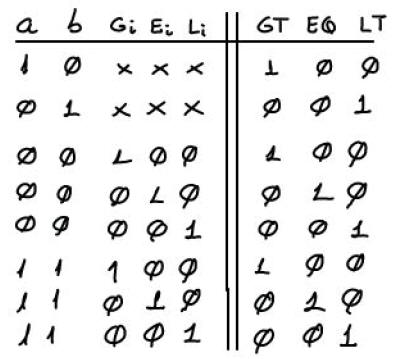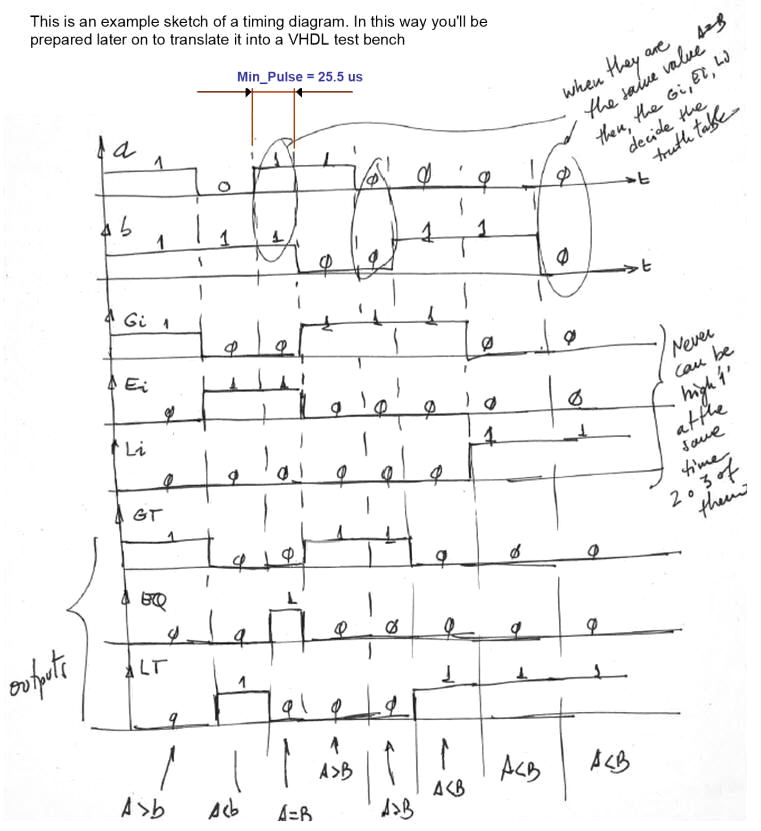﻿ Digital Circuits and Systems - Circuits i Sistemes Digitals (CSD) - EETAC - UPCBachelor's Degree in Telecommunications Systems and in Network Engineering## Comp_1bit plan A: structural single-file VHDL

Structural design of a 1-bit expandable comparator (Plan A)

1. Specifications

Design a 1-bit expandable comparator Comb_1bit using a set of structural equations, plan A.

It has the symbol and truth table represented in Fig. 1. The truth table has been adapted from the standard 4-bit commercial comparator chip 74LS85, the datasheet of which can be used to figure out how to chain comparator components to obtain larger circuits of the same kind.Fig. 1. Symbol and truth table of a 1-bit comparator with extra signals for expandability.

In Fig. 2 there is a simple sketch that represents some values of the truth table in time. The timing diagram wll be used in section 4th for testing the circuit using a VHDL testbench. All the 32 combinations can be applied sequentially in time to complete the test.Fig. 2. Timing diagram where all the inputs are applied sequentially and hence, the output is predicted accordingly to the truth table.

2. Planning

The VHDL file will be named Comp_1bit.vhd and the project name and location will be: Comp_1bit_prj,  "L:\CSD\P3\Comp_1bit_A\(files...) "

Plan A. Structural using equations. Let's get the simplified equations SoP or PoS using Minilog. For instance, this is the Comp_1bit.tbl, where you can see that an incomplete table is described. Many output situations are not important because the input combinations will never be possible in normal conditions, and so, this don't care output situations will help to further simplify the equations:

GT = f(a, b, Gi, Ei, Li)

EQ = f(a, b, Gi, Ei, Li)

LT = f(a, b, Gi, Ei, Li)

The result from minilog: Comp_1bit.min

3. Developing the project using EDA tools

Plan A structural equations. This is the translation to VHDL Comp_1bit.vhd

Run a EDA project to synthesise the circuit. Fig. 3 shows an example of RTL schematic.Fig. 3. Example RTL when the project is developed  using equations (plan A4).

4. Testing and validating the design

An example test bench Comp_1bit_tb.vhd. Run the VHDL simulation EDA tool to obtain and discuss the timing diagram.  Remember that from the testing point of view, even if you have designed the Adder_1bit using several plans, you can use all the time the same testbench.Fig. 4. Example testbench showing results.

5. Report

Project report starting with the template sheets of paper, scanned figures, file listings, docx , pptx, or any other resources.